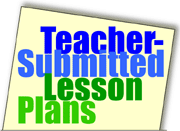Home >> A Tsl >> Archives >> 07 1 >> Math Dice Review Game

## Search formMath Dice Review Game

Subjects

• Arithmetic

• 3-5
• 6-8
• 9-12

Brief Description

This lesson can be used to review any math operation across the elementary to high school levels.

Objectives

Students review math operations by creating their own review problems using a specified number of dice and a whiteboard (or paper).

Keywords

math, game

• dice (The number of dice used in this game will vary depending on the math skill you wish to reinforce. If you wish for students to add, multiply, or subtract 4-digit numbers [for example, 4,768 2,582], students will need four dice per pair of students. If you wish students to divide a three-digit number by a two-digit number [for example, 360 divided by 18], they will need three dice.)
• one dry-erase board for each student (plain paper could be substituted)
• markers (if you use dry erase boards) or crayons/pencils (if you use paper)

The Lesson

Anticipatory Set

• Explain to the students that they will be working with partners to review recently taught math operations.
• Arrange students into pairs (one group of three if necessary).
• Supply each student with a dry-erase board (or paper). Give each pair of students a container with dice in it. (See Material Needed section above for the number of dice required.)

The Lesson
Once students are paired, introduce the directions of the game. Share with students that the dry-erase boards are to be used for their math calculations. In the sample lesson below, students will be asked to add three-digit numbers.

• One player in each pair begins the game by rolling the three dice in the container. That player makes up a three-digit number using the three numbers rolled. (For example, if the player rolled a 3, 2, and 6, he or she might create the number 263. Both students write that first number on their whiteboards/papers.
• The second player in each pair rolls the dice and creates another three-digit number. Both the students write that number on their whiteboards/papers.
• Once both players have written down the problem, they work on their own to solve it.
• When both students have done the math, they check their answers with one another. If their answers do not match, they solve again until they both have the same answer.
• The students roll again to create a new problem to solve

Assessment

Informally observe the activity to learn which students -- if any -- are having difficulty with the skill. If all students seem to have mastered the skill, they are ready to be tested on that skill.

Submitted By

Kaitlin Kelly, a student at the University of Scranton in Scranton, Pennsylvania

Education WorldÂ®